Application Notes

# Moku:Lab and Data Logging

Using the Data Logger to capture high speed mechanical impact

In this application note we describe the use of Moku:Lab‘s Data Logger to capture a high speed mechanical impact using a strain gauge. We are aiming to drop a steel ball onto an aluminium plate, measure the strain of this roughly 100 ms event, and observe any resulting mechanical oscillations

## Moku:Lab’s Data Logger instrument

Moku:Lab’s Data Logger instrument records time series voltages from 1 or 2 channels at rates from 1 sample per second up to 1 MSa/s. The data can be logged to RAM or a removeable SD card in a variety of formats including MATLAB’s .MAT file format. With sufficient storage, very lengthy logs of up to 240 hours can be created. The resulting logs can be shared to email or cloud services such as Dropbox. Moku:Lab’s Data Logger also includes an embedded waveform generator, useful for providing triggers or stimulus. Complete details on the Data Logger are available in the user manual .

### Experimental goal

We are aiming to drop a steel ball onto an aluminum plate and observe and record the strain of the impact and any resulting mechanical oscillations in the plate. We will use a strain gauge and strain gauge amplifier in conjunction with Moku:Lab’s Oscilloscope and Data Logger instruments.

### What is strain?

In mechanical test and measurement, strain is defined as the change in length of an object relative to its unstrained length. Strain (???? ) is defined thus:When an object is subject to compressive forces, its length reduces, while the other two dimensions will slightly increase. Equally, when an object is subject to tensile force, its length increases, while the other two dimensions will reduce (Figure 1).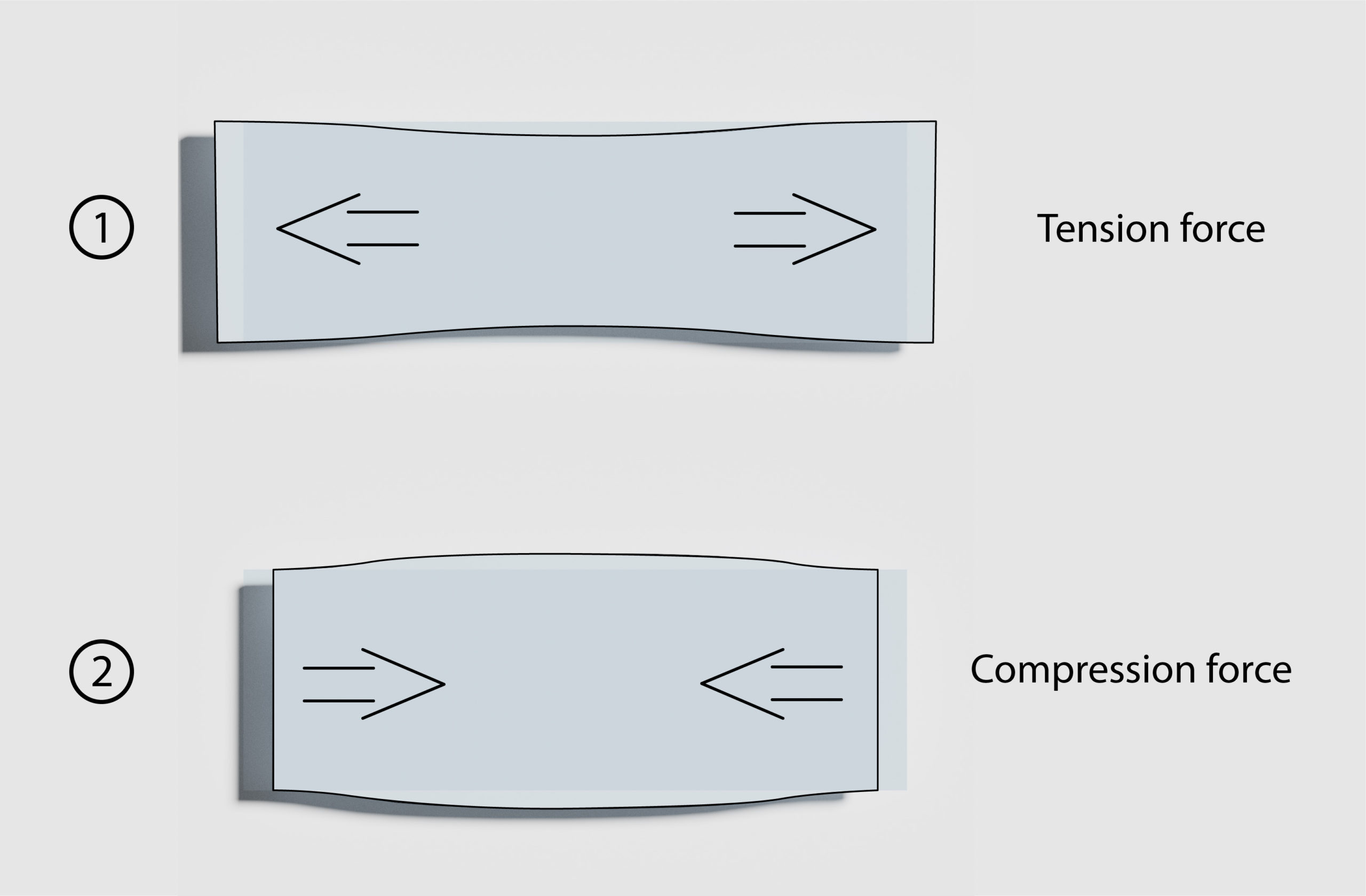Figure 1: Effect of tension and compression

When an object is bent, such as during an impact from above, the top surface will be in compression while the bottom surface is in tension (Figure 2).Figure 2: Effect of bending force

In practical situations, measured strain is very small and hence the unit-less measurement millistrain (10-3) or microstrain (10-6) is often used.

### Strain gauge background

A strain gauge is a mechanical-electrical sensor that allows us to measure mechanical strain. It relies upon the physical properties of conductance of an electrical conductor. The electrical resistance of a conductor of a given material is dependent upon the material’s conductivity and the conductor length and thickness. Resistance is defined by:w here R = resistance (Ω), l = length (m) and A (cross sectional area m2). ???? is the electrical resistivity of the material (Ω-m).

As a conductor is stretched, it will lengthen and narrow, both increasing the electrical resistance. Equally, as a conductor is compressed, it will shorten and thicken, both decreasing the electrical resistance. Thus, mechanical strain can be directly translated into electrical resistance.

Resistivity (????) of copper is 1.72 e-8 Ω-m; for a fine wire of diameter 0.1 mm in a compact size, of say, length 1 cm, the resistance is 22 milliohms. If we are aiming to measure millistrain, the measurement challenge is one of measuring very small resistance changes in the order of microohms. To make the resistance measurement simpler, a longer conductor length and ideally a material of higher resistivity is desirable.

A strain gauge implements this fine wire in a small, compact area by using a foil zig-zag pattern in order to maximize conductor length in a small footprint.Figure 3: Photograph of a strain gauge, 4mm x 6mm

In order to measure the resistance of the strain gauge, it is necessary to pass a current through the wire; this will cause some self-heating to occur. The temperature coefficient of copper, at room temperature, is 4.29 e-3 ppm/°C. So, for a modest increase of 10°C, the resistance changes 4.29 %. Clearly this negatively impacts the ability to measure strain accurately.

Commercially available strain gauges typically use the alloy constantan, a copper-nickel alloy.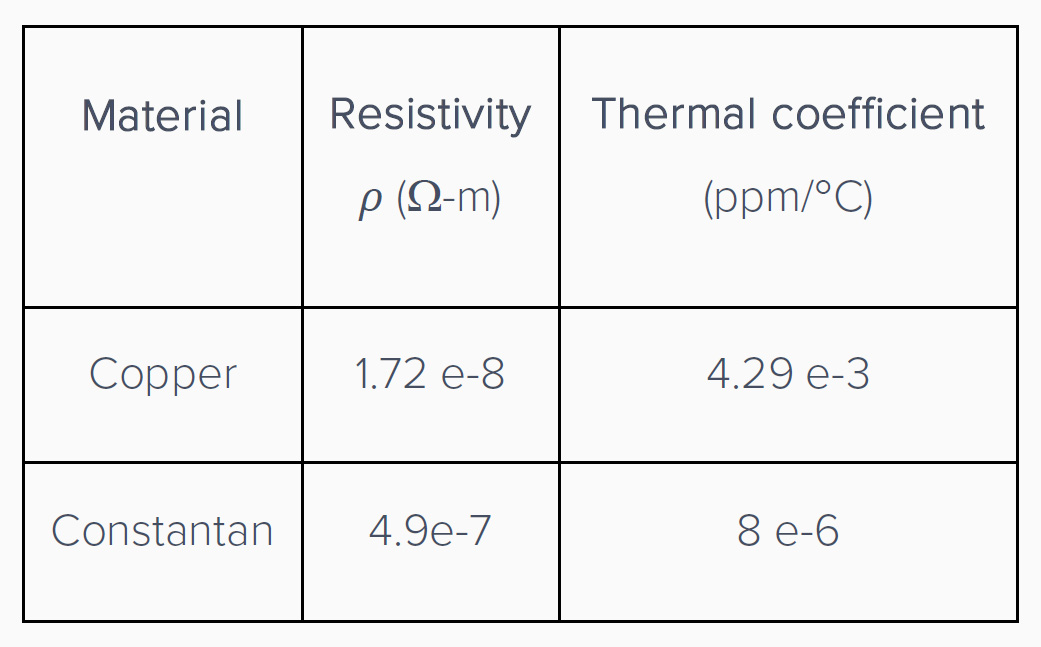Constantan has a resistivity (????) of 4.9e-7 (28 times higher than copper) and notably a temperature coefficient of 8 e-6 ppm/°C, >500 times more stable over temperature. A 10°C rise results in just 0.008 % change in resistance, a very useful benefit compared to copper.

Still, a small gauge made of a fine constantan zig-zag would have a typical unstrained resistance of just 100 Ω or so; and to measure milli-strain means we need to measure fractions of 1 Ω. Typical strain gauges have unstrained resistance of 120 Ω or 350 Ω.

### Wheatstone bridge

Small resistance changes are typically made with a Wheatstone bridge circuit. Such a circuit consists of two voltage dividers in parallel driven by an excitation voltage. A high impedance voltmeter measures the potential difference between the two voltage dividers. This effectively measures the resistance difference across the two arms and can result in very precise measurements of resistance changes. Further, the measurement does not vary due to voltage variations in the excitation source and provided the resistors are matched and of low temperature coefficients, this arrangement greatly reduces temperature effects.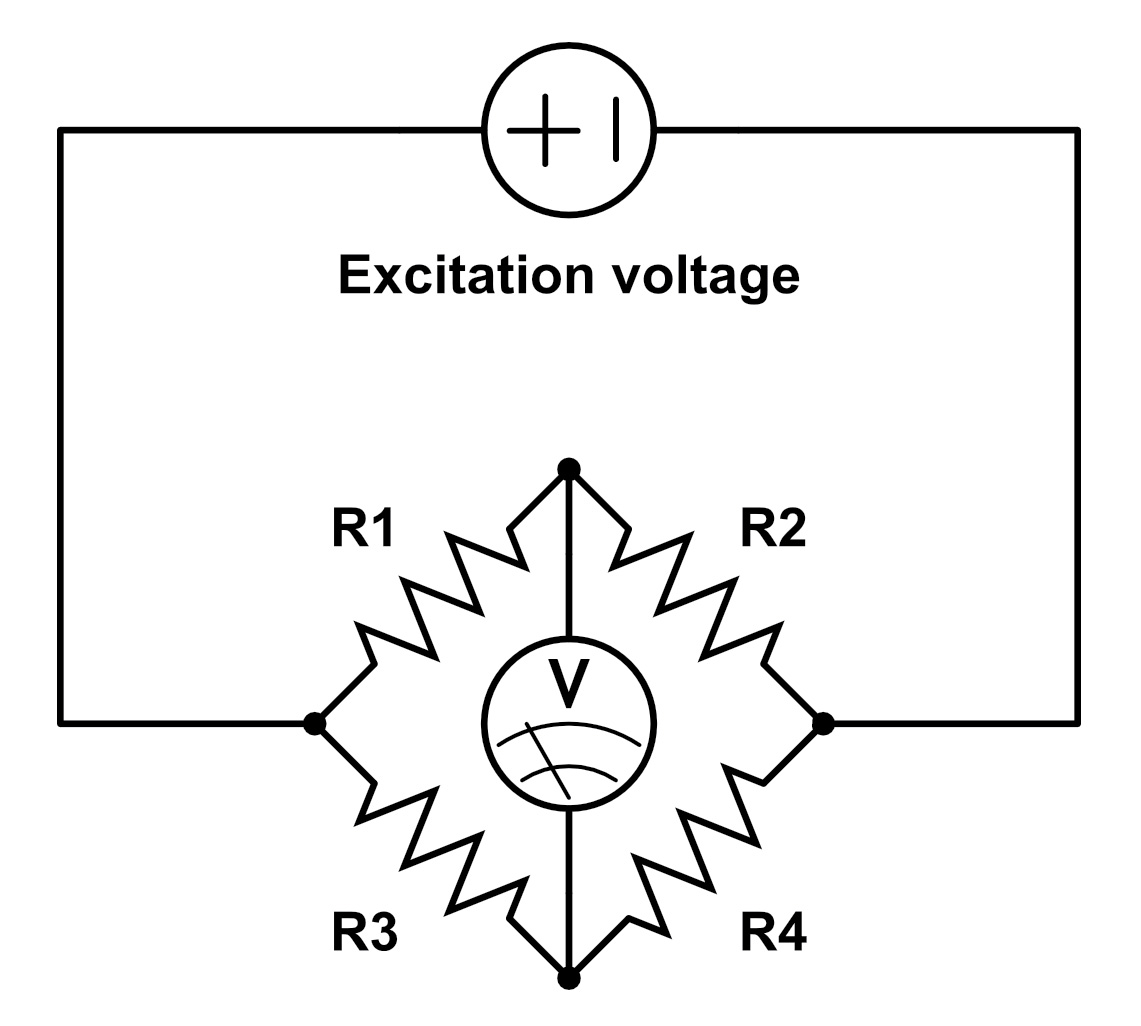Figure 4: Wheatstone bridge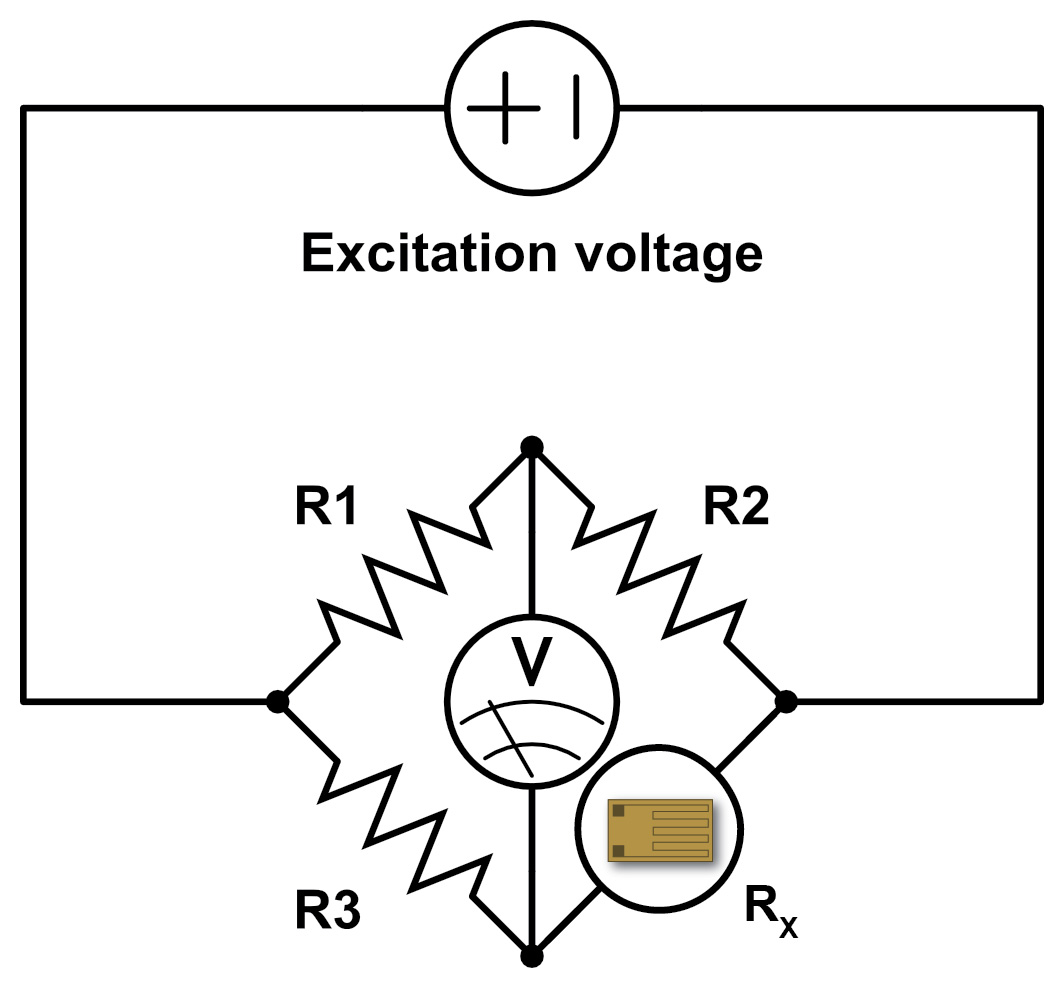Figure 5: Wheatstone bridge with strain gauge

Assuming the bridge is in balance, that is, there is 0 volts at the voltmeter (V), we can say:Assuming that the voltmeter is high impedance and negligible current flows through it, we can replace R4 with a strain gauge and state: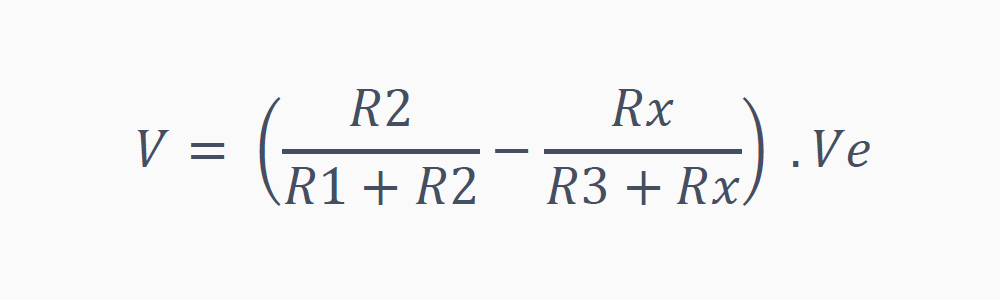Where Ve is the source excitation voltage and Rx the resistance of the strain gauge. Rearranging for Rx (see  for fuller discussion) gives: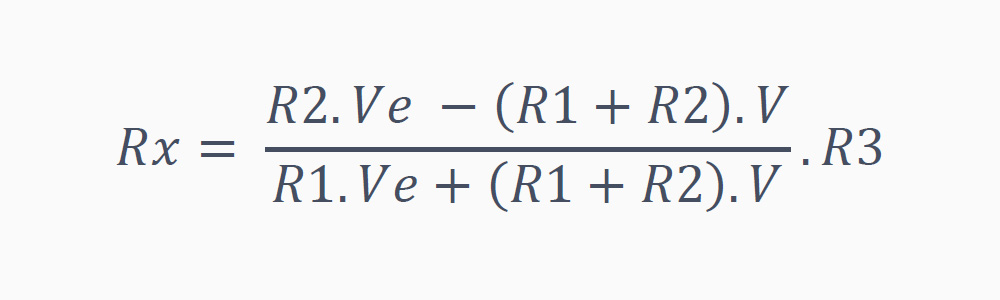Still, in order to measure millistrain we will be measuring millivolts and so we need a suitable amplifier. We used a readily available dedicated strain gauge amplifier  and configured it to 0.06 mV/V (equal to a gain of 16,667) to give a suitable output voltage range for Moku:Lab’s inputs (Figure 6).Figure 6: Strain gauge, amplifier and Moku:Lab

## Experimental setup

The experimental setup is shown in Figure 7. An aluminum plate is struck by a steel ball dropped from a height of 1 m. As the ball impacts the plate, we would expect to see an initial deflection of the bar (hence strain) followed by a brief, millisecond series of oscillation of the plate.Figure 7: Experimental setup

### Moku:Lab Data Logger setup

Moku:Lab’s Data Logger was used to capture the output of the strain gauge during the ball drop. The data logger was set to high speed (1 MSa/s) and the impact captured. The log file was shared directly from Moku:Lab via ethernet to a network connected PC as a “.MAT” format and then imported into MATLAB for viewing.

## Result and observations

Figure 8 shows Moku:Lab’s time series plot of the ball impact as captured on Moku:Lab’s Oscilloscope. This trace extends for approximately 11 ms and shows a large initial impact strain of around 1.5 ms followed by a series of oscillations of period of approximately 1.2 ms (or 833 Hz). Visually there appears to be some higher frequency components.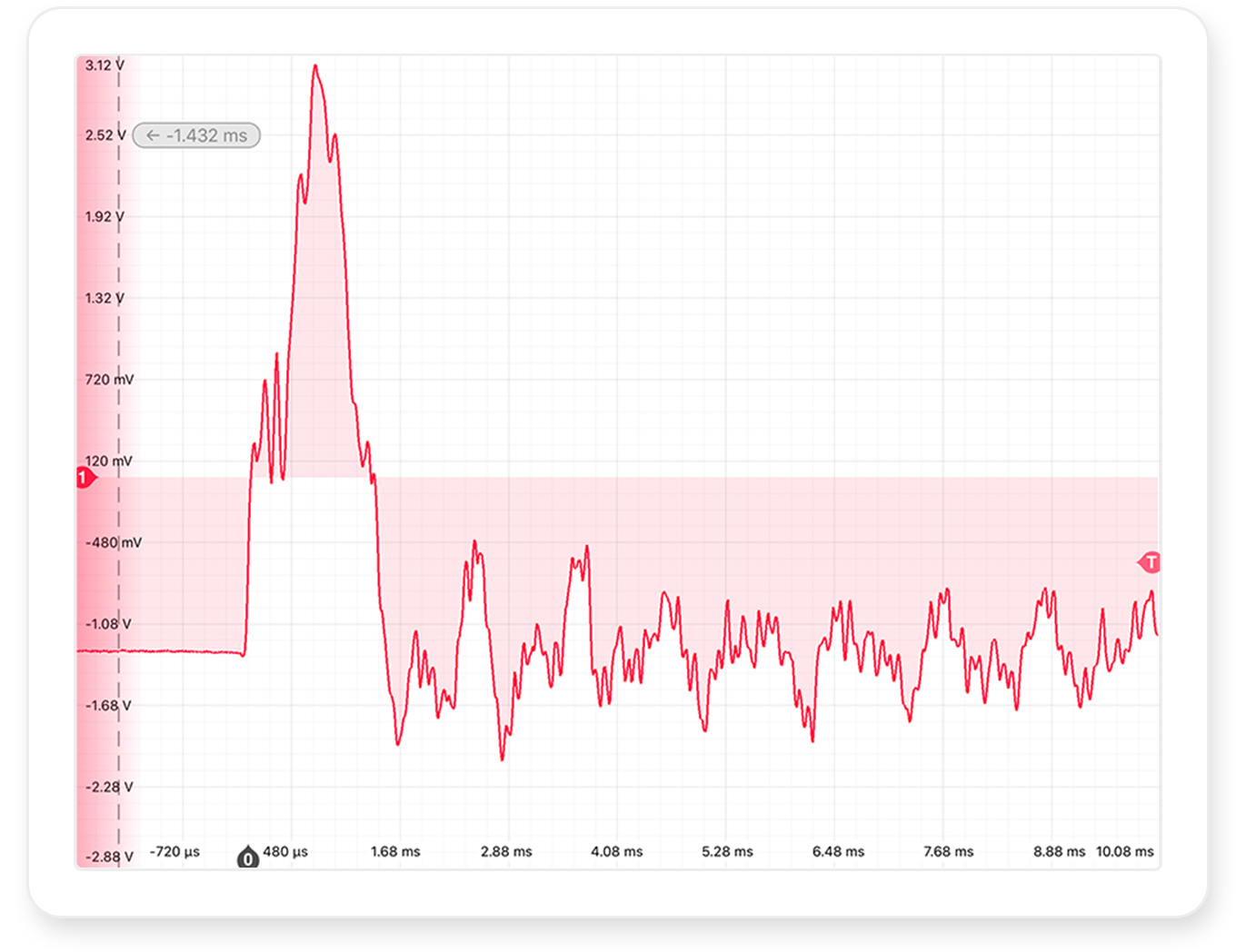Figure 8: Oscilloscope plot of impact

The .MAT file produced from Moku:Lab’s Data Logger was then imported into MATLAB and the longer time series is show in Figure 9.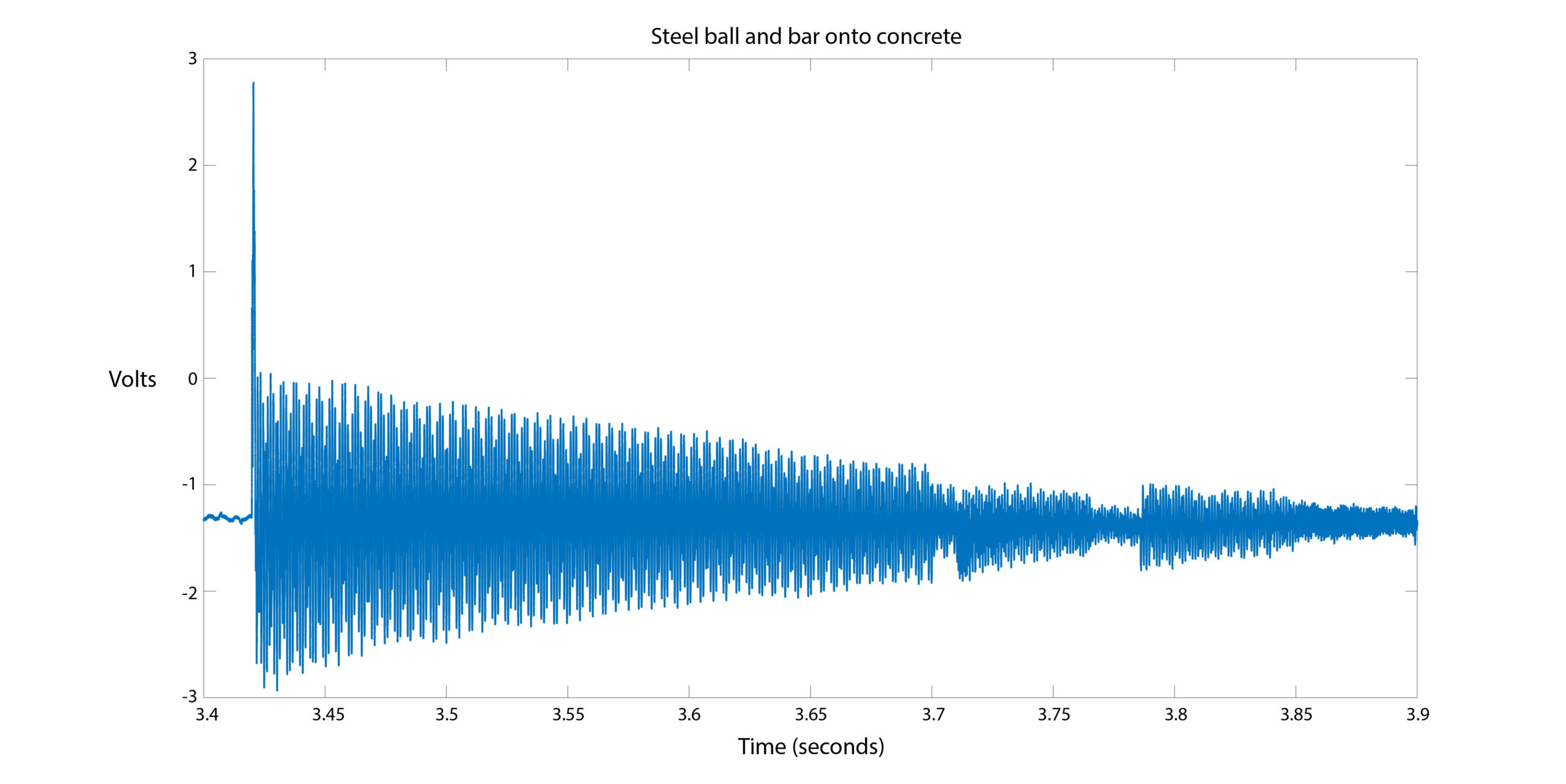Figure 9:Impact and oscillations from the Data Logger

This shows the large strain of the initial impact (3 V peak deviation), followed by more than 3.5 seconds of decaying oscillations. The abrupt amplitude changes at around 3.7 and 3.8 seconds correspond to the plate bouncing up and hitting the floor twice. Figure 10 shows the response from 3.45 s to 3.455 s and the oscillations previously noted on the oscilloscope.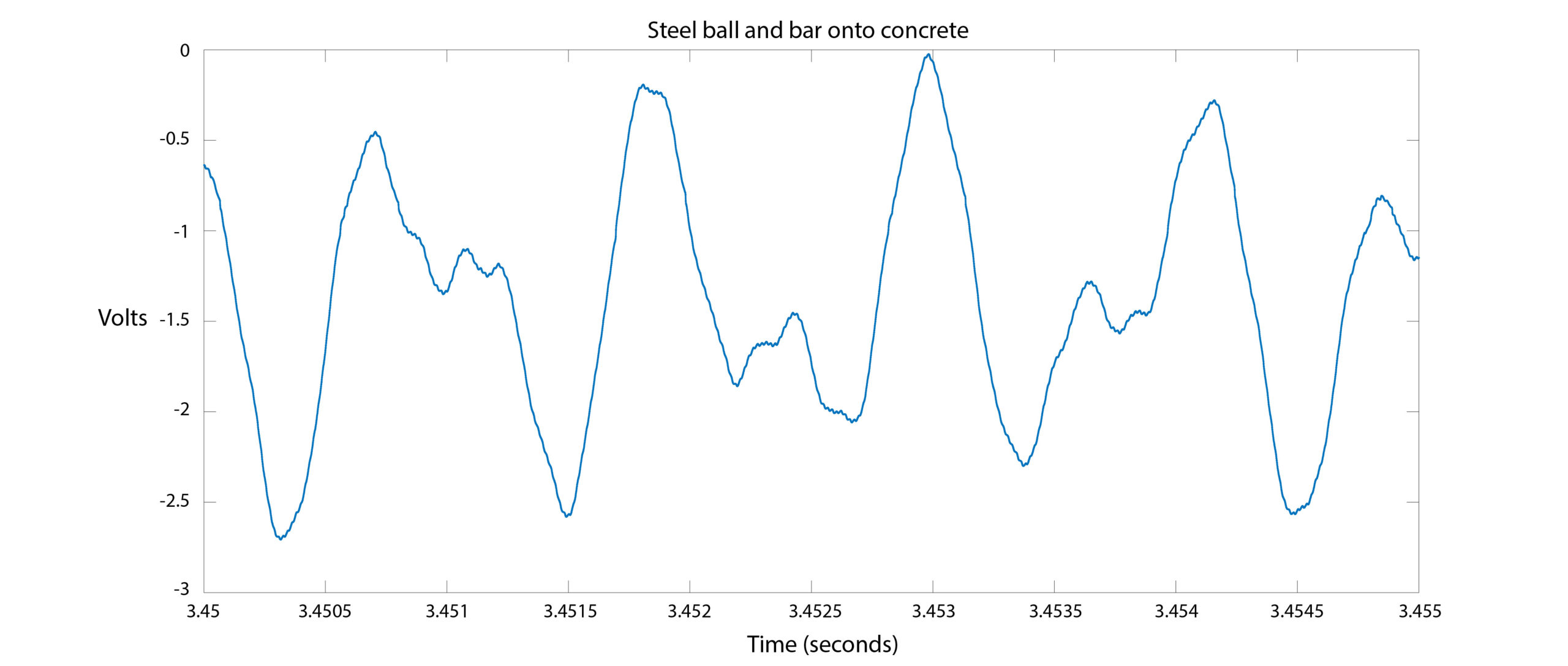Figure 10: Close up of oscillation from Data Logger

Since Moku:Lab allowed us to easily import the higher resolution time series in to MATLAB, we used MATLAB to produce a frequency plot (FFT) of the datalog. This is shown in Figure 11 with significant peaks of energy at 950 Hz and 1700 Hz.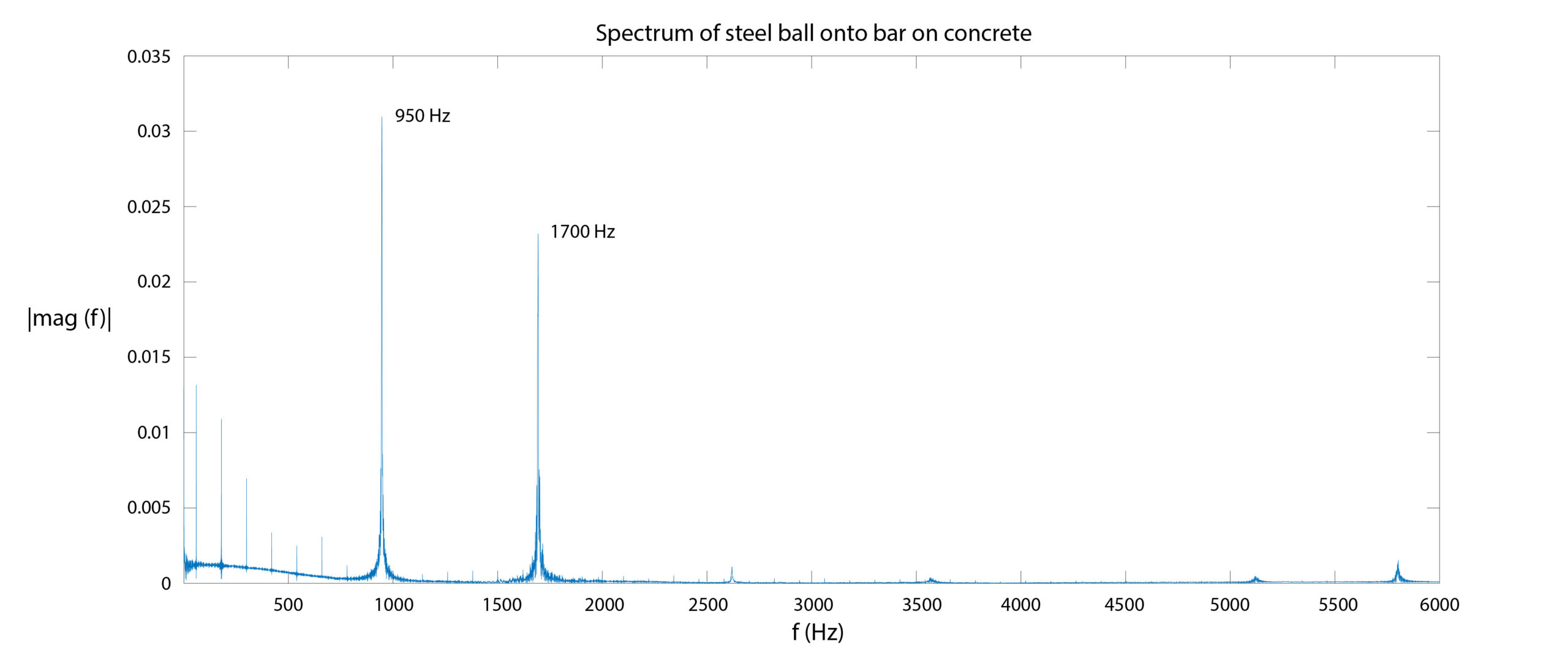Figure 11: FFT of impact datalog

The 950 Hz peak corresponds with the earlier estimate of 800 Hz from Moku:Lab Oscilloscope (Figure 8). There is very little oscillation energy above 1700 Hz.

## Summary

In this application note we have explained the basic concepts of mechanical strain and a common measurement method. We then used Moku:Lab’s Data Logger to record the strain of a mechanical impact of a steel ball dropped onto an aluminum plate.

Moku:Lab allowed for easy data sharing via Dropbox of a data log in MATLAB .MAT file format and the subsequent viewing of the time series and plotting of the frequency components.

Moku:Lab has MATLAB APIs and a future development of this experiment could be to automate the data collection and analysis entirely in MATLAB using Moku:Lab’s network connection.